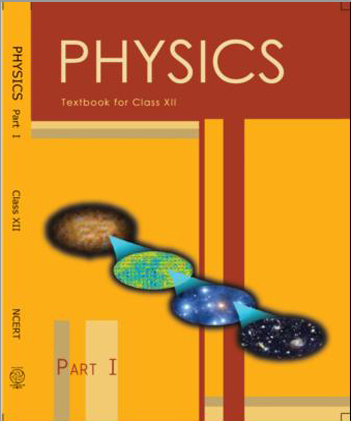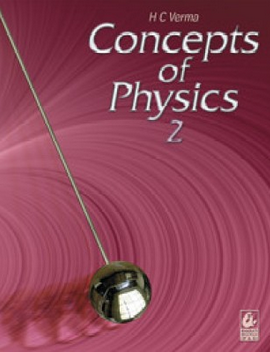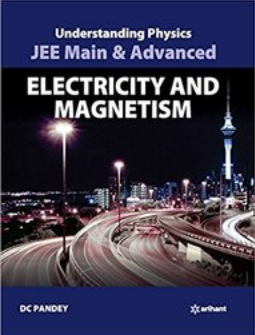# Magnetic Effects of Current and Magnetism (Weightage 5%)   Share

### Topics from Magnetic Effects of Current and Magnetism

• Biot Savart law and its application to current carrying circular loop. (17 concepts)
• Bar magnet as an equivalent solenoid, magnetic field lines (62 concepts)
• Ampere's law and its applications to infinitely long current carrying straight wire and solenoid. (4 concepts)
• Current loop as a magnetic dipole and its magnetic dipole moment. (27 concepts)
• Force on a moving charge in uniform magnetic and electric fields, Cyclotron (27 concepts)
• Force on a current-carrying conductor in a uniform magnetic field. (13 concepts)
• The force between two parallel current-carrying conductors-definition of the ampere. (2 concepts)
• Earth's magnetic field and magnetic elements. Para-, dia- and ferro- magnetic substances. (48 concepts)
• Torque experienced by a current loop in uniform magnetic field (10 concepts)
• Moving coil galvanometer, its current sensitivity, and conversion to ammeter and voltmeter. (11 concepts)
• Magnetic susceptibility and permeability, Hysteresis (15 concepts)
• Electromagnets and permanent magnets. (5 concepts)
• Biot-Savart Law and its applications (4 concepts)
• Ampere's law and its applications (5 concepts)
• Motion of a charged particle in uniform magnetic and electric fields (5 concepts)
• Magnetism and matter (8 concepts)
• Magnetic force on a current carrying conductor (2 concepts)
• Torque on a current loop, Magnetic Dipole (3 concepts)
• Moving coil galvanometer (1 concepts)

### Important Books for Magnetic Effects of Current and Magnetism

•••Exams
Articles
Questions